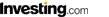兑换数量:       选择货币 南非兰特(ZAR) 人民币(CNY) 港元(HKD) 台币(TWD) 欧元(EUR) 美元(USD) 英镑(GBP) 澳元(AUD) 韩元(KRW) 日元(JPY) 中非法郎(XAF) -------A---------- 安哥拉宽扎(AOA) 阿尔巴尼亚列克(ALL) 阿尔及利亚第纳尔(DZD) 阿根廷比索(ARS) 阿鲁巴岛弗罗林(AWG) 澳元(AUD) 埃及镑EGP) 埃塞俄比亚比尔(ETB) 澳门元(MOP) 阿曼里亚尔(OMR) 阿联酋迪拉姆(AED) -------B----------- 巴哈马元(BSD) 巴林第纳尔(BHD) 巴巴多斯元(BBD) 白俄罗斯卢布(BYR) 伯利兹元(BZD) 百慕大元(BMD) 不丹卢比(BTN) 玻利维亚诺(BOB) 博茨瓦纳普拉(BWP) 巴西里亚伊(BRL) 保加利亚列瓦(BGN) 布隆迪法郎(BIF) 冰岛克朗(ISK) 巴基斯坦卢比(PKR) 巴拿马巴尔博亚(PAB) 巴布亚新几内亚基那(PGK) 巴拉圭瓜拉尼(PYG) 波兰兹罗提(PLN) --------C---------- 朝鲜圆(KPW) --------D---------- 多哥非洲共同体法郎(XOF) 丹麦克朗(DKK) 多米尼加比索(DOP) --------E---------- 俄罗斯卢布(RUB) --------F---------- 佛得角埃斯库多(CVE) 福克兰群岛镑(FKP) 斐济元(FJD) 菲律宾比索(PHP) --------G---------- 格鲁吉亚拉里(GEL) 刚果中非共同体法郎(XAF) 哥伦比亚比索(COP) 哥斯达黎加科朗(CRC) 古巴比索CUP) 格林纳达东加勒比元(XCD) 冈比亚达拉西(GMD) 圭亚那元(GYD) --------H---------- 海地古德(HTG) 洪都拉斯伦皮拉(HNL) 哈萨克斯坦腾格(KZT) --------J---------- 柬埔寨利尔斯(KHR) 加拿大元(CAD) 捷克克朗(CZK) 吉布提法郎(DJF) 几内亚法郎(GNF) 津巴布韦元(ZWD) --------K---------- 科摩罗法郎(KMF) 克罗地亚库纳(HRK) 肯尼亚先令(KES) 科威特第纳尔(KWD) 卡塔尔利尔(QAR) --------L---------- 老挝基普(LAK) 拉脱维亚拉图(LVL) 黎巴嫩镑(LBP) 莱索托洛提(LSL) 利比里亚元(LRD) 利比亚第纳尔(LYD) 立陶宛里塔斯(LTL) 列斯荷兰盾(ANG) 罗马尼亚新列伊(RON) 卢旺达法郎(RWF) --------M---------- 孟加拉塔卡(BDT) 马其顿第纳尔(MKD) 马拉维克瓦查(MWK) 马来西亚林吉特(MYR) 马尔代夫卢非亚(MVR) 毛里塔尼亚乌吉亚(MRO) 毛里求斯卢比(MUR) 墨西哥比索(MXN) 摩尔多瓦列伊(MDL) 蒙古图格里克(MNT) 摩洛哥道拉姆(MAD) 缅甸元(MMK) 秘鲁索尔(PEN) 莫桑比克美提卡(MZM) --------N---------- 纳米比亚元(NAD) 尼泊尔卢比(NPR) 尼加拉瓜科多巴(NIO) 尼日利亚奈拉(NGN) 挪威克朗(NOK) 南非兰特(ZAR) --------R---------- 瑞典克朗(SEK) 瑞士法郎(CHF) --------S---------- 萨尔瓦多科朗(SVC) 萨摩亚塔拉(WST) 圣多美多布拉(STD) 沙特阿拉伯里亚尔(SAR) 塞舌尔法郎(SCR) 塞拉利昂利昂(SLL) 所罗门群岛元(SBD) 索马里先令(SOS) 斯里兰卡卢比(LKR) 圣赫勒拿群岛磅(SHP) 斯威士兰里兰吉尼(SZL) --------T---------- 土耳其新里拉(TRY) 太平洋法郎(XPF) 坦桑尼亚先令(TZS) 泰国铢(THB) 汤加潘加(TOP) 特立尼达和多巴哥元(TTD) 突尼斯第纳尔(TND) --------W---------- 文莱元(BND) 危地马拉格查尔(GTQ) 乌克兰赫夫米(UAH) 乌拉圭新比索(UYU) 瓦努阿图瓦图(VUV) 越南盾(VND) --------X---------- 匈牙利福林(HUF) 新西兰元(NZD) 新加坡元(SGD) 叙利亚镑(SYP) --------Y---------- 印度卢比(INR) 印度尼西亚卢比(盾)(IDR) 伊朗里亚尔(IRR) 伊拉克第纳尔(IQD) 以色列镑(ILS) 牙买加元(JMD) 约旦第纳尔(JOD) 也门里亚尔(YER) --------Z---------- 智利比索(CLP) 直布罗陀镑(GIP) 赞比亚克瓦查(ZMK) ---------贵金属--------- 铜价盎司(XCP) 金价盎司(XAU) 钯价盎司(XPD) 铂价盎司(XPT) 银价盎司(XAG) 选择货币 人民币(CNY) 南非兰特(ZAR) 港元(HKD) 台币(TWD) 欧元(EUR) 美元(USD) 英镑(GBP) 澳元(AUD) 韩元(KRW) 日元(JPY) 中非法郎(XAF) -------A---------- 安哥拉宽扎(AOA) 阿尔巴尼亚列克(ALL) 阿尔及利亚第纳尔(DZD) 阿根廷比索(ARS) 阿鲁巴岛弗罗林(AWG) 澳元(AUD) 埃及镑EGP) 埃塞俄比亚比尔(ETB) 澳门元(MOP) 阿曼里亚尔(OMR) 阿联酋迪拉姆(AED) -------B----------- 巴哈马元(BSD) 巴林第纳尔(BHD) 巴巴多斯元(BBD) 白俄罗斯卢布(BYR) 伯利兹元(BZD) 百慕大元(BMD) 不丹卢比(BTN) 玻利维亚诺(BOB) 博茨瓦纳普拉(BWP) 巴西里亚伊(BRL) 保加利亚列瓦(BGN) 布隆迪法郎(BIF) 冰岛克朗(ISK) 巴基斯坦卢比(PKR) 巴拿马巴尔博亚(PAB) 巴布亚新几内亚基那(PGK) 巴拉圭瓜拉尼(PYG) 波兰兹罗提(PLN) --------C---------- 朝鲜圆(KPW) --------D---------- 多哥非洲共同体法郎(XOF) 丹麦克朗(DKK) 多米尼加比索(DOP) --------E---------- 俄罗斯卢布(RUB) --------F---------- 佛得角埃斯库多(CVE) 福克兰群岛镑(FKP) 斐济元(FJD) 菲律宾比索(PHP) --------G---------- 格鲁吉亚拉里(GEL) 刚果中非共同体法郎(XAF) 哥伦比亚比索(COP) 哥斯达黎加科朗(CRC) 古巴比索CUP) 格林纳达东加勒比元(XCD) 冈比亚达拉西(GMD) 圭亚那元(GYD) --------H---------- 海地古德(HTG) 洪都拉斯伦皮拉(HNL) 哈萨克斯坦腾格(KZT) --------J---------- 柬埔寨利尔斯(KHR) 加拿大元(CAD) 捷克克朗(CZK) 吉布提法郎(DJF) 几内亚法郎(GNF) 津巴布韦元(ZWD) --------K---------- 科摩罗法郎(KMF) 克罗地亚库纳(HRK) 肯尼亚先令(KES) 科威特第纳尔(KWD) 卡塔尔利尔(QAR) --------L---------- 老挝基普(LAK) 拉脱维亚拉图(LVL) 黎巴嫩镑(LBP) 莱索托洛提(LSL) 利比里亚元(LRD) 利比亚第纳尔(LYD) 立陶宛里塔斯(LTL) 列斯荷兰盾(ANG) 罗马尼亚新列伊(RON) 卢旺达法郎(RWF) --------M---------- 孟加拉塔卡(BDT) 马其顿第纳尔(MKD) 马拉维克瓦查(MWK) 马来西亚林吉特(MYR) 马尔代夫卢非亚(MVR) 毛里塔尼亚乌吉亚(MRO) 毛里求斯卢比(MUR) 墨西哥比索(MXN) 摩尔多瓦列伊(MDL) 蒙古图格里克(MNT) 摩洛哥道拉姆(MAD) 缅甸元(MMK) 秘鲁索尔(PEN) 莫桑比克美提卡(MZM) --------N---------- 纳米比亚元(NAD) 尼泊尔卢比(NPR) 尼加拉瓜科多巴(NIO) 尼日利亚奈拉(NGN) 挪威克朗(NOK) 南非兰特(ZAR) --------R---------- 瑞典克朗(SEK) 瑞士法郎(CHF) --------S---------- 萨尔瓦多科朗(SVC) 萨摩亚塔拉(WST) 圣多美多布拉(STD) 沙特阿拉伯里亚尔(SAR) 塞舌尔法郎(SCR) 塞拉利昂利昂(SLL) 所罗门群岛元(SBD) 索马里先令(SOS) 斯里兰卡卢比(LKR) 圣赫勒拿群岛磅(SHP) 斯威士兰里兰吉尼(SZL) --------T---------- 土耳其新里拉(TRY) 太平洋法郎(XPF) 坦桑尼亚先令(TZS) 泰国铢(THB) 汤加潘加(TOP) 特立尼达和多巴哥元(TTD) 突尼斯第纳尔(TND) --------W---------- 文莱元(BND) 危地马拉格查尔(GTQ) 乌克兰赫夫米(UAH) 乌拉圭新比索(UYU) 瓦努阿图瓦图(VUV) 越南盾(VND) --------X---------- 匈牙利福林(HUF) 新西兰元(NZD) 新加坡元(SGD) 叙利亚镑(SYP) --------Y---------- 印度卢比(INR) 印度尼西亚卢比(盾)(IDR) 伊朗里亚尔(IRR) 伊拉克第纳尔(IQD) 以色列镑(ILS) 牙买加元(JMD) 约旦第纳尔(JOD) 也门里亚尔(YER) --------Z---------- 智利比索(CLP) 直布罗陀镑(GIP) 赞比亚克瓦查(ZMK) ---------贵金属--------- 铜价盎司(XCP) 金价盎司(XAU) 钯价盎司(XPD) 铂价盎司(XPT) 银价盎司(XAG)

 外汇行情由 Investing.com 中文站 提供。Flash 图表由Investing.com 中文站 提供。